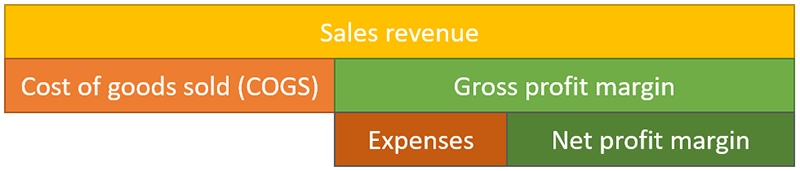# Margin Calculator

Use this margin calculator to easily calculate your profit margin (operating margin), your gross or net profit or the revenue required to achieve a given margin. Enter the cost and either the total revenue, the gross/net profit or the gross/net margin percentage to calculate the remaining two.

\$
\$
\$
%
Share calculator:

Embed this tool:
get code

## How to use the Margin Calculator?

• profit, gross/net profit margin and markup given cost and gross/net revenue.
• revenue, gross/net profit margin and markup given cost and gross/net profit.
• revenue, profit and markup given the cost and the profit margin.

Simply enter the cost and the other business metric depending on the desired output and press "Calculate". You can copy/paste the results easily using the clipboard icon next to each value. Knowing your margin and the markup needed to achieve a given net or gross profit can help run a business more successfully.

## What is gross profit margin?

The gross profit margin (a.k.a. operating margin, operating profit margin, operating income margin, EBIT margin) is a key business performance metric indicating the profitability of a company, product or investment project. It is great for internal comparisons of one period versus another, identifying trends in profitability, as well as comparisons to businesses of similar industries, niches, sizes and age.

The profit margin is so key as it communicates the percentage of total revenue converted to operating profits (before tax profits). It is often used by investors as an efficiency ratio or percentage metric as it is a proxy for potential dividend payouts, reinvestment potential and overall solvency. The result is a measurement of what proportion of a company's revenue is left over, before taxes and other indirect costs (such as bonuses, interest rate payments), after paying for variable production costs such as wages, source materials, contractors, etc. A higher operating margin means that the company has less financial risk as it is able to face fixed cost expenses with greater ease.## What is net profit margin?

Whereas a gross profit margin is the margin before taxes and interest, it does account for depreciations and ammortization in the cost part of the equation. A net profit margin would include deductions of taxes and interest payments. In order to calculate it, include taxes and interest in the value for the "Cost" input field in our profit margin calculator.

So, for net margin the Cost variable includes both COGS (Cost of goods sold), expenses (Operating expenses and various expenses) and interest and taxes, whereas for gross margin the Cost is just COGS and expenses.

## Gross margin formula

The formula for gross margin is:

Margin = Operating income / Revenue

Operating income is also called "operating profit" whereas revenue is total value of sales and it is usually tightly tied to the selling price. In many cases the total costs and revenue are known and what is sought is the operating income and margin. In such circumstances the following profit margin formula is more suitable, which is why it is used in our g margin calculator:

Margin = (Revenue - Cost) / Revenue

Both input values are in the relevant currency while the resulting profit margin is a percentage (gross margin percentage, e.g. 10%) arrived at after multiplying the result by 100. Note that our profit margin calculator does not do any currency conversions, so make sure you input the values in the same currency.

## Net margin formula

To compute the net profit margin, simply use the gross margin equation above, but include taxes and interest payments in the cost variable. For gross margin Cost means just the cost of goods sold and operating expenses whereas for net margin it is cost of goods sold and expenses plus taxes plus interest payments on debt capital.

## How to calculate your profit margin?

Knowing the formula above, you should start with estimating the cost of production, which includes all variable costs of producing the goods or services the business sells. If calculating for a past period, you would already know the gross revenue that was made by selling the goods or services. Simply plug in the numbers in formula #2 above and you will get the result. For example, if the costs are \$100,000 and the revenue is \$120,000 the equation becomes: Margin = (120,000 - 100,000) / 120,000 = 20,000 / 120,000 = 1/6 which is the margin ratio telling you that for every 6 dollars in sales the business pockets 1 dollar in profit. To convert to percentage, multiply by 100: 1/6 * 100 = 16.67% operating profit margin.

If you know only the cost and the profit, simply add the two together to get the revenue, then substitute in equation #2 again. If what you want to calculate is the profit and/or revenue required to achieve a given margin, then simply input the cost and the margin percentage in our calculator and it will handle the rest.

## What is a good gross profit margin?

While a profit margin calculation is useful in itself, some might need more context to interpret the numbers. Generally, a good profit margin should allow the business to cover its variable and fixed expenses and turn a profit with which to compensate the capital owners for their risk (time preference). This is individual to every business or investment project and what is a "good profit margin" depends very much on the options it is compared with, as well as the estimated risk.

#### Cite this calculator & page

If you'd like to cite this online calculator resource and information as provided on the page, you can use the following citation:
Georgiev G.Z., "Margin Calculator", [online] Available at: https://www.gigacalculator.com/calculators/profit-margin-calculator.php URL [Accessed Date: 06 Jun, 2023].